Difference between revisions of "Faber-Schauder system"

A system of functions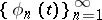on an intervalconstructed as follows using an arbitrary countable sequence of points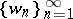,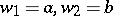, that is everywhere dense in this interval. Set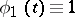on. The function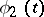is linear onsuch that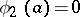,. If, then one dividesinto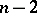parts by the points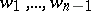and one chooses the interval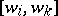,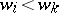, that contains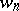. Then one sets,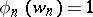, and extends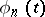linearly to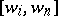and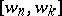. Outside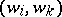one setsequal to zero.
In the case when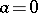,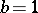, andis the sequence of all dyadic rational points in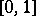, enumerated in the natural way (that is, in the order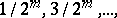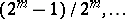), the system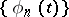(denoted by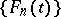) first appeared in the work of G. Faber . He considered it (with another normalization) as the system of indefinite integrals of the Haar system supplemented by the function that is identically equal to one. In the general case, the construction ofwas carried out by J. Schauder, and so a Faber–Schauder system is also called a Schauder system.
The systemis a basis of the space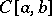of all continuous functionsonwith norm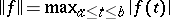(see ,  or ). If one applies the Schmidt orthogonalization process to the Faber systemon, the Franklin system is obtained.